### Home > MC1 > Chapter 4 > Lesson 4.1.1 > Problem4-10

4-10.
1. . Express each of the following lengths in feet, Homework Help ✎

1. 24 inches

2. 18 inches

3. 9 inches

4. 2 yards

5. 3 inches

6. 2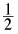yards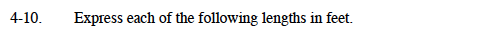Remember that 12 inches are equal to 1 foot and 3 feet are equal to 1 yard. These conversions will come in handy!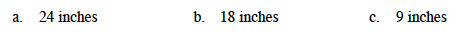Try drawing a diagram of how many inches are in a foot to compare to each of these measures.

For instance, James converted 36 inches to feet by drawing the diagram at right. He could see that there are 3 groups of 12 inches in 36 inches, so that means 36 inches is equal to 3 feet!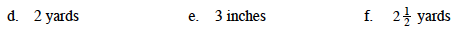If one yard is 3 feet, how many feet are in 2 yards? Can you use multiplication? Why would that make sense?

$3\text{ inches }=\frac{1}{4}\text{ feet.}$

Don't forget to show your work.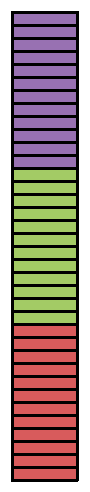$2\frac{1}{2}\text{ yards }=7\frac{1}{2}\text{ feet.}$